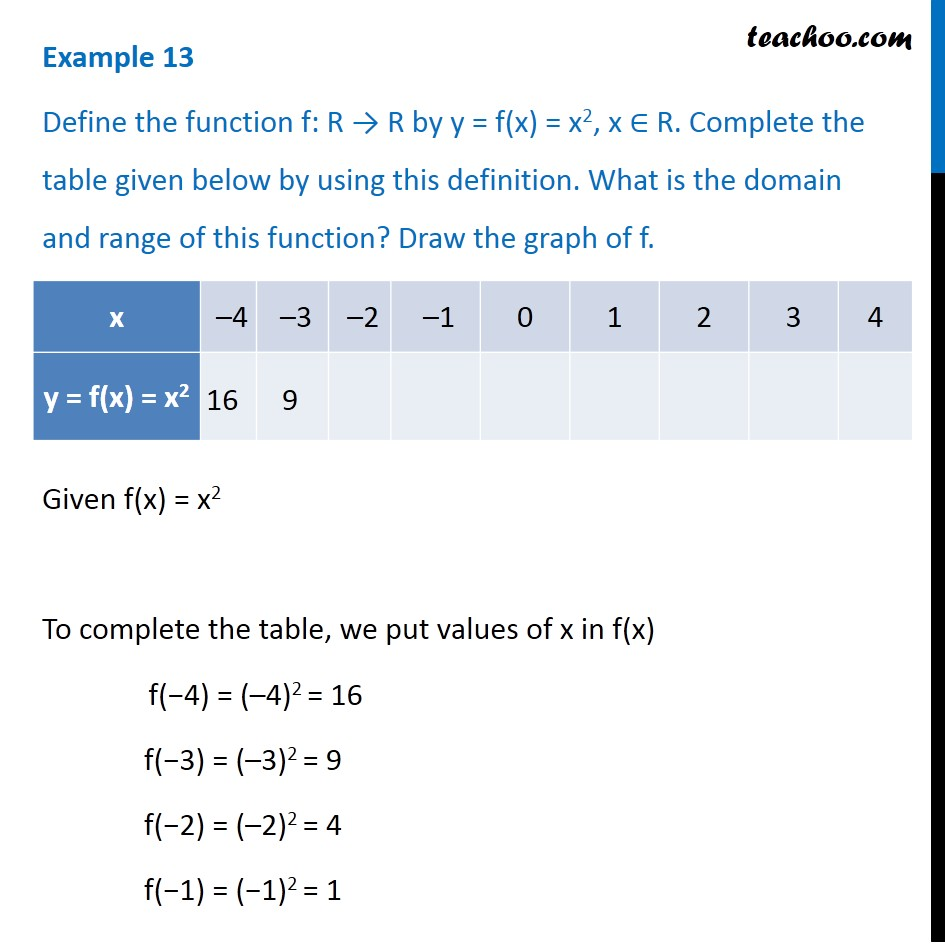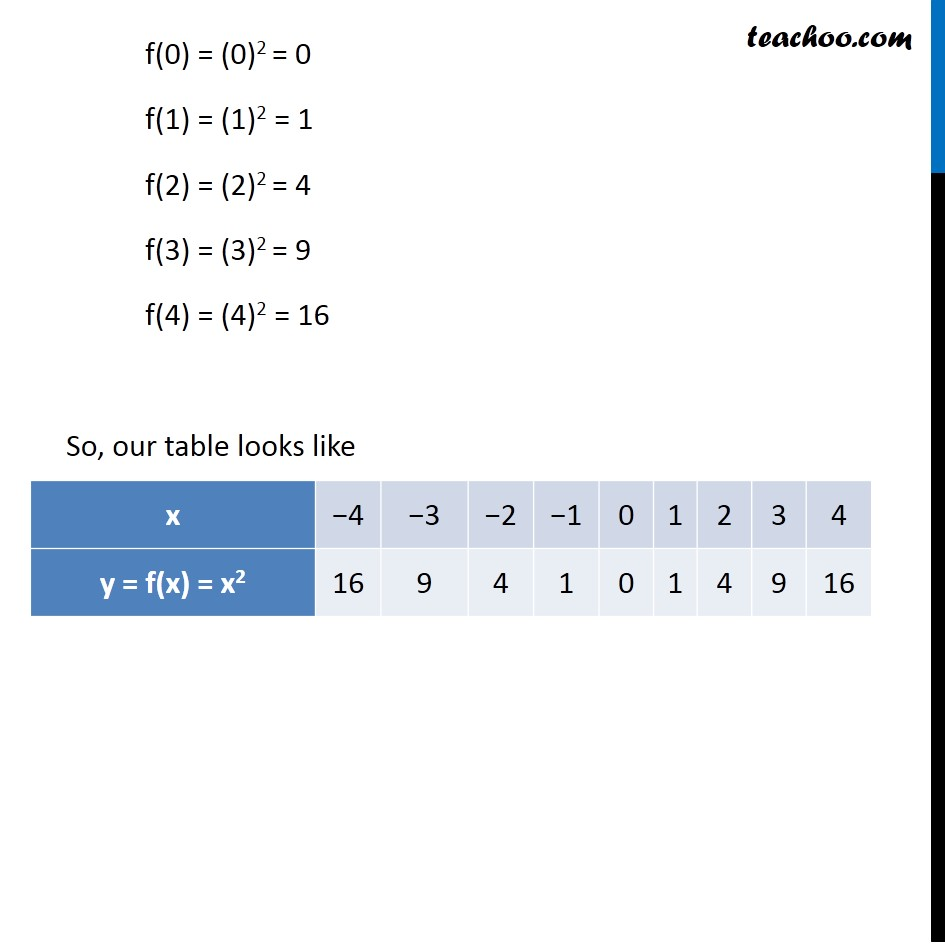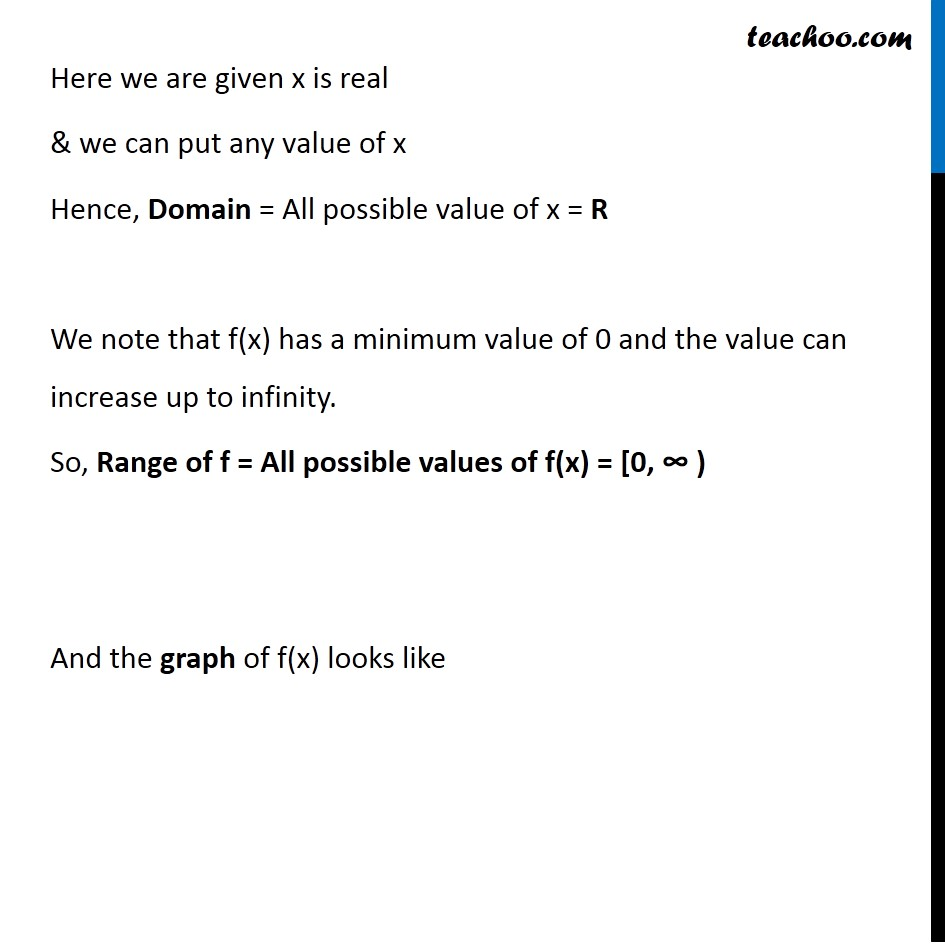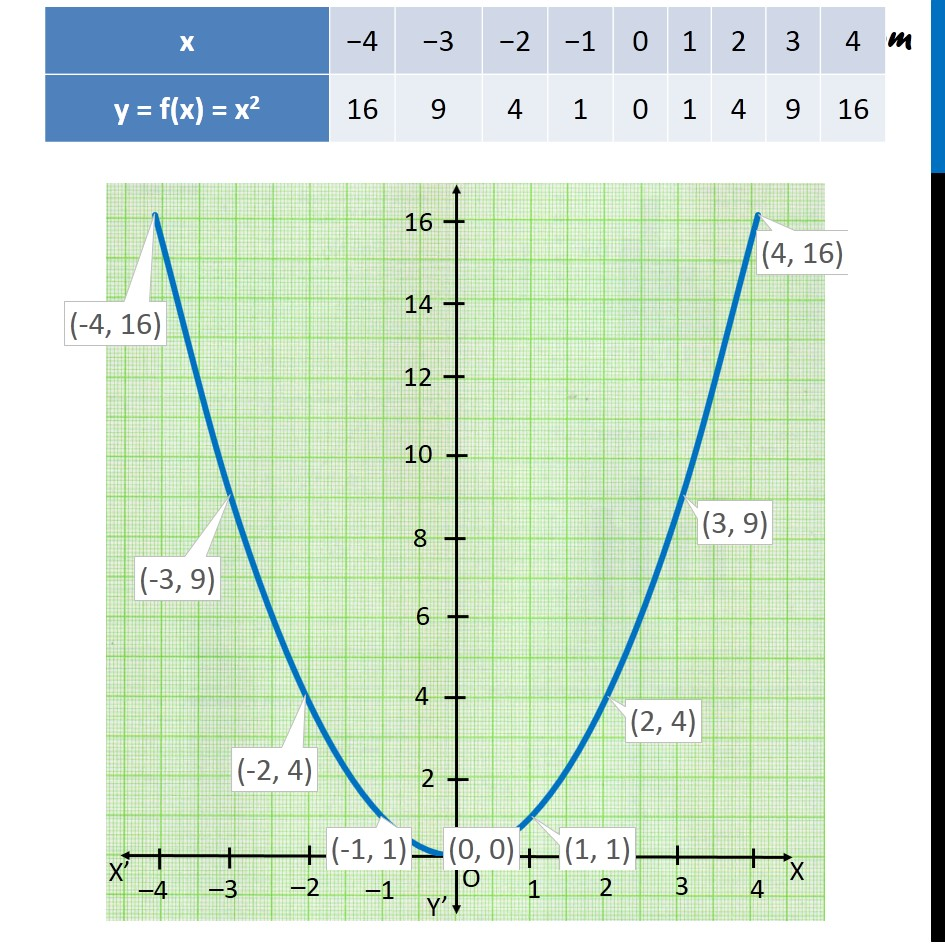Examples

Chapter 2 Class 11 Relations and Functions
Serial order wiseLearn in your speed, with individual attention - Teachoo Maths 1-on-1 Class

### Transcript

Example 13 Define the function f: R → R by y = f(x) = x2, x ∈ R. Complete the table given below by using this definition. What is the domain and range of this function? Draw the graph of f. Given f(x) = x2 To complete the table, we put values of x in f(x) f(−4) = (–4)2 = 16 f(−3) = (–3)2 = 9 f(−2) = (–2)2 = 4 f(−1) = (−1)2 = 1 f(0) = (0)2 = 0 f(1) = (1)2 = 1 f(2) = (2)2 = 4 f(3) = (3)2 = 9 f(4) = (4)2 = 16 So, our table looks like Here we are given x is real & we can put any value of x Hence, Domain = All possible value of x = R We note that f(x) has a minimum value of 0 and the value can increase up to infinity. So, Range of f = All possible values of f(x) = [0, ∞ ) And the graph of f(x) looks like